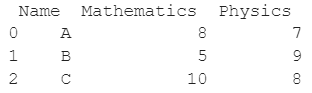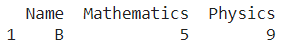# How to Select A Row Of Pandas Dataframe By Integer Index?

A DataFrame is the primary data structure of the Pandas library and is commonly used for storing and working with tabular data. A common operation that could be performed on such data is selecting a row of pandas dataframe by integer index in order to extract information from it.

To start working with Pandas, we first need to import it in Python code :

### Python 3 Code :

``import pandas as pd``

## Running Example

Let us understand this operation with the help of an example. Consider the following DataFrame containing 3 students with names A, B, and C and their corresponding marks (out of 10) for two subjects, Mathematics and Physics.Code snippet for generating the above DataFrame :

### Python 3 Code :

``````import pandas as pd

# Dictionary for our data
data = {'Name' : ['A', 'B', 'C'], 'Mathematics' : [8, 5, 10], 'Physics' : [7, 9, 8]}

# DataFrame for the dictionary
df = pd.DataFrame(data)

# Printing the DataFrame
print(df)``````

Here, data is a dictionary we created to initialize the DataFrame. For this, we use the DataFrame() function of the Pandas library which takes the dictionary as an argument and returns the required DataFrame.

Now, let’s say we need to extract the information of one particular student and we know only the integer index for this, i.e select a particular row of the dataframe using integer index.

The resulting DataFrame would look like this :Let us look at different ways of performing this operation on a given Pandas DataFrame :

## Selecting A Row Of Pandas Dataframe By Integer Index Using the dataframe.loc Property

This method is pretty straightforward and is the most commonly used one. In this method, we use the dataframe.loc property with the required integer index, 1 here, passed as a parameter.

Remember that the index starts from 0 and not 1 so for the second row the required index is 1 and not 2. The changes are not made in place so we need to reassign the column.

Let us look at the Python code and corresponding output for this method:

### Python 3 Code :

``````import pandas as pd

# Dictionary for our data
data = {'Name' : ['A', 'B', 'C'], 'Mathematics' : [8, 5, 10], 'Physics' : [7, 9, 8]}

# DataFrame for the dictionary
df = pd.DataFrame(data)

# Selecting the second row using integer inde
df = df.loc[]

# Printing the new dataframe
print(df)``````

Output :## Selecting A Row Of Pandas Dataframe By Integer Index Using the dataframe.iloc property

This method is pretty straightforward and is the most commonly used one. In this method, we use the dataframe.iloc property with the required integer index, 1 here, passed as a parameter.

Remember that the index starts from 0 and not 1 so for the second row the required index is 1 and not 2. The changes are not made in place so we need to reassign the column.

Let us look at the Python code and corresponding output for this method:

### Python 3 Code :

``````import pandas as pd

# Dictionary for our data
data = {'Name' : ['A', 'B', 'C'], 'Mathematics' : [8, 5, 10], 'Physics' : [7, 9, 8]}

# DataFrame for the dictionary
df = pd.DataFrame(data)

# Selecting the second row using integer index
df = df.iloc[]

# Printing the new dataframe
print(df)``````

Output :## Conclusion

In this topic, we have learned to select a row of Pandas dataframe by integer index from an existing Pandas DataFrame, following a running example of test scores of students in different subjects, thus giving us an intuition of how this concept could be applied in real-world situations. Feel free to reach out to info.javaexercise@gmail.com in case of any suggestions.

Trending